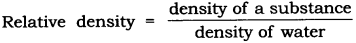Thursday , June 30 2022# 9th Class (CBSE) Science: Gravitation

#### Question: What is the value of gravitational constant G: (1) On the earth, (2) On the moon.

1.  6.67 × 10-11  Nm2 kg2
2. 6.67 × 10-11  Nm2 kg2

#### Question: Which of the Kepler’s laws of planetary motion led Newton to establish the inverse square rule for gravitational force between to bodies?

Answer:  Third Law, T2 ∝ r3

#### Question: Which force is responsible for holding the solar system together.

Answer: Gravitational force of the Sun.

#### Question: What is the weight of a 1 kilogram mass on the earth? (g = 9.8m/s2)

1 kg × 9.8 m/s2  (W = mg)
= 9.8 N

#### Question: What is the S.I. unit of pressure?

Answer: The S.I. unit of pressure = N/m2 = Pascal.

#### Question: Define thrust.

Answer: The net force exerted by a body in a particular direction is called thrust.

#### Question: Define pressure.

Answer: The force exerted per unit area is called pressure.

#### Question: Why is it easier to swim in sea water than in river water?

Answer: The density of sea water is more due to dissolved salts in it as compared to the density of river water. Hence the buoyant force exerted on the swimmer by the sea water is more which helps in floating and makes swimming easier.

#### Question: Why a truck or a motorbike has much wider tyres?

Answer: The pressure exerted by it can be distributed to more area, and avoid the wear and tear of tyres.

#### Question: Why are knives sharp?

Answer: To increase the pressure, area is reduced,

As pressure ∝ 1/Area hence the pressure or force exerted on a body increases.

#### Question: Why is the wall of dam reservoir thicker at the bottom?

Answer: The pressure of water in dams at the bottom is more, to withstand this pressure the dams have wider walls.

#### Question: Why do nails have pointed tips?

Answer: The force exerted when acts on a smaller area, it exerts larger pressure. So the nails have pointed tips.

#### Question: While swimming why do we feel light?

Answer: The swimmer is exerted by an upward force by water, this phenomenon is called buoyancy and it makes the swimmer feel light.

#### Question: Define density and give its unit.

Answer: The density of a substance is defined as mass per unit volume. Its unit is kg/m3.

#### Question: What is relative density?

Answer: The relative density of a substance is the ratio-of its density to that of water.#### Question: A ship made of iron does not sink but the iron rod sinks in water, why?

Answer: The iron rod sinks due to high density and less buoyant force exerted by the water on it, but in case of ship the surface area is increased, the upthrust experienced by the body is more. So it floats on water

## Sources of Energy: 10th Science Chapter 14

Class: 10th Class Subject: Science Chapter: Chapter 14: Sources of Energy Quiz: – Questions MCQs: …